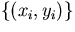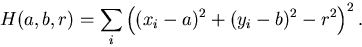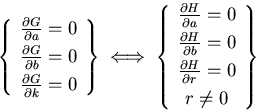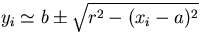MAT 331, Spring 98
Project 1: Least-Squares Fitting
Due 3 March 1998

This project, like all in this class, have a significant component which is expository in nature. Please pay attention to organization, sentence structure, and so on. You will be graded on both the quality of your mathematical exposition and on the correctness of your computer work. You should treat this like a paper, rather than a typical homework assignment. A good paper should be complete and self-contained, going over all the necessary background material.

Part 1.    In this part, you are given a collection of pointswhich you believe approximate a circle. You should find the best fit'' circle to these points using least squares. In your write-up, you should not only find the circle, but explain clearly what you are doing at each step and why.

As we saw in class, the desired circle corresponds to the minimum of the functionWhile maple can find the critical points of this equation directly, you should do the following:

Let k=a2+b2-r2 to obtain a new functional G(a,b,k) . Taking the partials with respect to the parameters a , b , and k gives three linear expressions in the three variables, so G has a unique critical point, which is the desired minimum. Give a complete explanation of this change of variables.

In your project, you should give a proof that the circle which gives a minimum for G always corresponds to the minimum for H . You could do this by showing thatIn your paper, you should discuss this case in full detail, and give an example. Use the routine circle_pts() to generate some data points which approximate a circle.

Optionally, you could also discuss other possible means of measuring the distance between a collection of points and a circle. For example, what would be the disadvantage to using the usual'' method of least squares to fit the relationship?

Part 2.    Suppose the data points (xi,yi) are assumed to approximate a line, but both the x -values and the y -values are viewed as approximate. In this case, it makes sense to minimize the sum of the squares of the shortest distance to the line, rather than the vertical distance. That is, if (x,y) are points on the line and (xi,yi) are data points, you should vary the line to minimize the sum of the quantities (x-xi)2 + (y-yi)2 . Note that there are several (equivalent) ways to represent the line and/or compute the distance. You should, of course, choose whichever seems best to you.

You are to derive a least-squares fitting method for this case, and illustrate it by an example. Compare the line you find in this example to the one given by usual'' least squares. Do they give essentially the same line? When do they differ? Explain.

Note that it is not possible to obtain linear equations; there will always be more than one critical point. Only one of them corresponds to the minimum- what is the geometric interpretation of the other one? Be sure to describe the mathematics of what you are doing in sufficient detail. In particular, you will need to derive a formula for the smallest distance between a point (xi, yi) and a line y=mx+b . Do not merely present the formula; derive it and explain your derivation.

Note: While it is acceptable to discuss the problems with each other and how to solve them, the write-up you present must be your own. Also note that each person should have a different collection of data, since it is generated at random. If you have significant discussions with others, it would be nice to acknowledge who they are. You can produce your paper in any word-processor you like (or even write it by hand, if your writing is quite legible), or as a heavily annotated maple worksheet. In all cases, you should include the relevant graphics and maple commands, as needed. These, of course, cannot be handwritten, but may be inserted via cut and paste'' if you like. Each part of the project should hang together as a single document, rather than as some discussion with a maple session tagged on at the end.

Scott Sutherland
2/19/1998